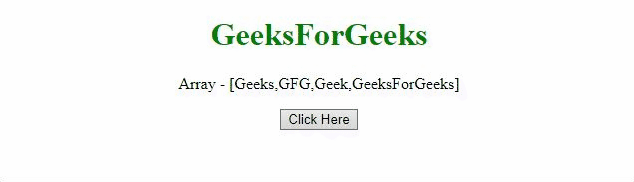Related Articles
Delete the first element of array without using shift() method in JavaScript
• Last Updated : 27 Apr, 2020

Given an array containing some array elements and the task is to remove the first element from the array and thus reduce the size by 1. We are going to perform shift() method operation without actually using it with the help of JavaScript.
There are two approaches that are discussed below:

Approach 1: We can use the splice() method that is used to get the part of the array.

• Example:
 ```<``html``>`` ` `<``head``>``    ``<``title``>``        ``Delete first element from the array without``        ``using shift() operation in JavaScript``    `````` ` `<``body` `style``=``"text-align:center;"``>``     ` `    ``<``h1` `style``=``"color:green;"``>``        ``GeeksForGeeks``    ```` ` `    ``<``p` `id``=``"GFG_UP"``>`` ` `    ``<``button` `onclick``=``"myGFG()"``>``        ``Click Here``    ````     ` `    ``<``p` `id``=``"GFG_DOWN"``>``     ` `    ``<``script``>``        ``var arr = ['Geeks', 'GFG', 'Geek', 'GeeksForGeeks'];``        ``var up = document.getElementById("GFG_UP");``        ``up.innerHTML = "Array - [" + arr + "]";``        ``var down = document.getElementById("GFG_DOWN");``        ``function myGFG() {``            ``arr.splice(0, 1);``            ``down.innerHTML = "elements of array = [" + arr + "]";``        ``}``    `````` ` ``
• Output:Approach 2: We can use the filter() method to filter out the element at index 0.

• Example:
 ```<``html``>`` ` `<``head``>``    ``<``title``>``        ``Delete first element from the array without``        ``using shift() operation in JavaScript``    `````` ` `<``body` `style``=``"text-align:center;"``>``     ` `    ``<``h1` `style``=``"color:green;"``>``        ``GeeksForGeeks``    ````     ` `    ``<``p` `id``=``"GFG_UP"``>`` ` `    ``<``button` `onclick``=``"myGFG()"``>``        ``Click Here``    ````     ` `    ``<``p` `id``=``"GFG_DOWN"``>`` ` `    ``<``script``>``        ``var arr = ['Geeks', 'GFG', 'Geek', 'GeeksForGeeks'];``        ``var up = document.getElementById("GFG_UP");``        ``up.innerHTML = "Array - [" + arr + "]";``        ``var down = document.getElementById("GFG_DOWN");``        ``function removeFirst(element, index) {``            ``return index > 0;``        ``}``        ``function myGFG() {``            ``arr = arr.filter(removeFirst);``            ``down.innerHTML = "elements of array = [" ``                            ``+ arr + "]";``        ``}``    `````` ` ``
• Output:My Personal Notes arrow_drop_up